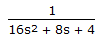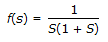Courses

# Test: Instrumentation & Process Control - 7

## 25 Questions MCQ Test Instrumentation and Process Control | Test: Instrumentation & Process Control - 7

Description
This mock test of Test: Instrumentation & Process Control - 7 for Electrical Engineering (EE) helps you for every Electrical Engineering (EE) entrance exam. This contains 25 Multiple Choice Questions for Electrical Engineering (EE) Test: Instrumentation & Process Control - 7 (mcq) to study with solutions a complete question bank. The solved questions answers in this Test: Instrumentation & Process Control - 7 quiz give you a good mix of easy questions and tough questions. Electrical Engineering (EE) students definitely take this Test: Instrumentation & Process Control - 7 exercise for a better result in the exam. You can find other Test: Instrumentation & Process Control - 7 extra questions, long questions & short questions for Electrical Engineering (EE) on EduRev as well by searching above.
QUESTION: 1

Solution:
QUESTION: 2

Solution:
QUESTION: 3

### A constant volume gas thermometer employing __________ is used to measure sub-zero (i.e., < 0o C) temperature.

Solution:
QUESTION: 4

Routh test

Solution:
QUESTION: 5

pH meter has

Solution:
QUESTION: 6

The transfer function of a second order system is

Solution:
QUESTION: 7

The transfer function of a process is. If a step change is introduced into the system, then the response will be

Solution:
QUESTION: 8

Which of the following thermocouples is the most suitable for measuring a temperature of about 1600oC in an oxidizing atmosphere ?

Solution:
QUESTION: 9

Bode diagram are generated from output response of the system subjected to which of the following input?

Solution:
QUESTION: 10

Mercury thermometer can be used to measure the temperature upto __________ oC.

Solution:
QUESTION: 11

Which of the following is not a differential pressure flow meter ?

Solution:
QUESTION: 12

Vapour actuated pressure spring thermometer does not require ambient temperature compensation. Ambient temperature compensation is provided in gas or liquid expansion pressure spring thermometer by

Solution:
QUESTION: 13

Working principle of mercury in glass thermometer is based on volumetric expansion of mercury with increase in temperature. Which of the following undergoes minimum volumetric expansion for a given temperature change ?

Solution:
QUESTION: 14

Bourdon gauges are used for measuring pressure (kg/cm2 )

Solution:
QUESTION: 15

A photo electric device in which the resistance of the metal is directly proportional to the light striking on it, is known as photo-conductive cell. Photoelectric transducers are used for the measurement of those parameters, which can be used to produce variation in

Solution:
QUESTION: 16

A typical example of a physical system with under damped characteristic is a

Solution:
QUESTION: 17

An aneroid barometer measures the __________ pressure.

Solution:
QUESTION: 18

A bolometer is

Solution:
QUESTION: 19

Thermal wells are used in the temperature measurement to

Solution:
QUESTION: 20

The offset introduced by proportional controller with gain Kcin response of first order system can be reduced by

Solution:
QUESTION: 21

Thermal conductivity based continuous flue gas analyser makes use of varying thermal conductivity of the constituents of flue gases. Which of the following constituents of flue gases has the maximum thermal conductivity ?

Solution:
QUESTION: 22

The inverse Laplace transform of the functionis

Solution:
QUESTION: 23

Emf generated in a thermocouple depends on the temperature

Solution:
QUESTION: 24

Working principle of mercury in glass thermometer is based on the __________ of mercury with increase in temperature.

Solution:
QUESTION: 25

The Laplace transform of a sinusoidal function (eg; sin at) is represented by the curve

Solution: